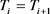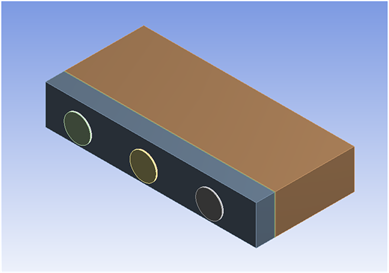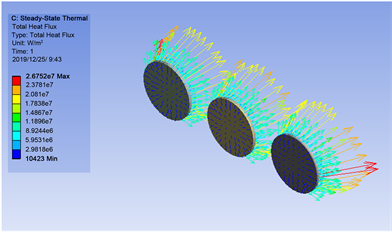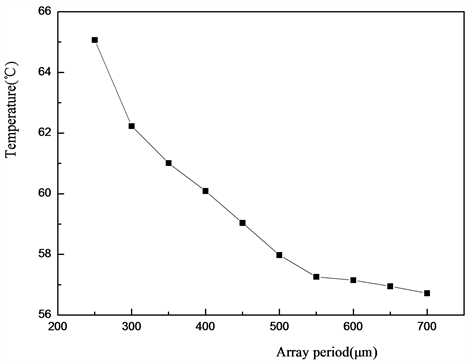﻿ 微盘腔半导体激光器阵列热分析

# 微盘腔半导体激光器阵列热分析Thermal Analysis of Micro-Disk Cavity Semiconductor Laser Array

Abstract: Micro-cavity lasers have been widely studied because of their advantages such as simple geometry, small size, low threshold, dynamic mode operation and easy integration with other electronic components. For micro-disk cavity lasers that have achieved directional output, the output power can be effectively increased by array distribution. This paper uses ANSYS finite element analysis software to simulate the heat dissipation model of micro-cavity semiconductor laser. On the basis of exploring the heat dissipation mode of the micro-cavity laser array, the temperature variation rule of the chip is obtained by changing the cycle of the laser array. The results show that a micro-cavity laser array with a radius of 100 µm can achieve a better heat dissipation effect when the array period is 550 µm.

1. 引言

2. 激光器阵列稳态热模拟

2.1. 模型建立

$W={P}_{out}+Q$ (1)

$\eta =\frac{{P}_{out}}{W}$ (2)

$q=\frac{Q}{V}=\frac{{P}_{out}\left(\frac{1-\eta }{\eta }\right)}{N×\text{d}×S}$ (3)

${-k\frac{\partial T}{\partial n}|}_{s}=0$ (4)(5)(6)Table 1. Modeling parameters in ANSYS simulationFigure 1. Micro-disk cavity semiconductor laser array model

2.2. 结果与讨论Figure 2. Heat flux vector diagram of micro-cavity laser arrayFigure 3. (a) 250 μm; (b) 450 μm; (c) 550 μm; (d) 700 μm array periodic heat distribution cloud mapFigure 4. Curves of periodic and temperature variations of arrays

3. 结论

NOTES

*通讯作者。

 刘学智. 光电子与激光技术的发展与应用[C]//天津市电子工业协会. 天津市电子工业协会2019年年会论文集.天津市电子工业协会: 天津市电子工业协会, 2019: 2.

 周旭彦, 赵少宇, 马晓龙, 等. 低垂直发散角高亮度光子晶体半导体激光器[J]. 中国激光, 2017, 44(2): 020-101.

 Tian, Z., Li, S.L., Kiravittaya, S., Xu, B.R., Tang, S.W., Zhen, H.L., Lu, W. and Mei, Y.F. (2018) Selected and Enhanced Single Whispering-Gallery Mode Emission from a Mesostructured Nanomembrane Microcavity. Nano Letters, 1021, 117-125.

 Papp, S.B., Beha, K., Del Haye, P., et al. (2014) Microresonator Frequency Comb Optical Clock. Optica, 1, 10-14.
https://doi.org/10.1364/OPTICA.1.000010

 Mccall, S.L., Levi, A.F.J., Slusher, R.E. et al. (1992) Whispering-gallery Mode Microdisk Lasers. Applied Physics Letters, 60, 289-291.
https://doi.org/10.1063/1.106688

 Shang, L., Liu, L. and Xu, L. (2008) Highly Collimated Laser Emission from a Peanut-Shaped Microcavity. Applied Physics Letters, 92, 071111-071111-3.
https://doi.org/10.1063/1.2839383

 Lee, S.-Y., Kurdoglyan, M., Rim, S., et al. (2004) Resonance Patterns in a Stadium-Shaped Microcavity. Physical Review A, 70, 23-89.
https://doi.org/10.1103/PhysRevA.70.023809

 陈天奇, 张普, 彭勃, 张宏友, 吴的海. 封装对大功率半导体激光器阵列热应力及Smile的影响[J]. 光子学报, 2018, 47(6): 129-139.

 曹宇, 李克彬, 窦洋, 杨国涛, 朱婉莹, 郑权. 大功率半导体激光器散热研究综述[J]. 科学技术创新, 2019(18): 19-20.

 姜晓光, 赵英杰, 吴志全. 基于ANSYS半导体激光器热特性模拟与分析[J]. 长春理工大学学报(自然科学版), 2010, 33(1): 41-43.

 Saeed Moaveni, 莫维尼, 王崧, 等. 有限元分析:ANSYS理论与应用[M]. 北京: 电子工业出版社, 2013.

Top